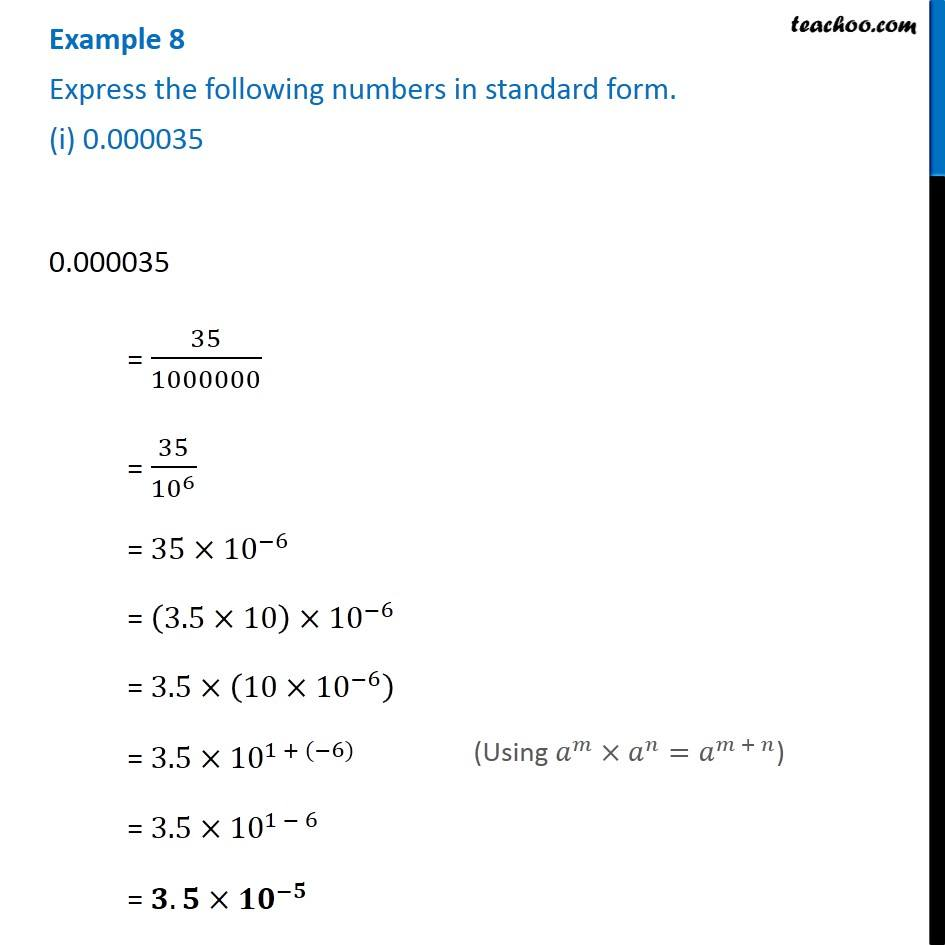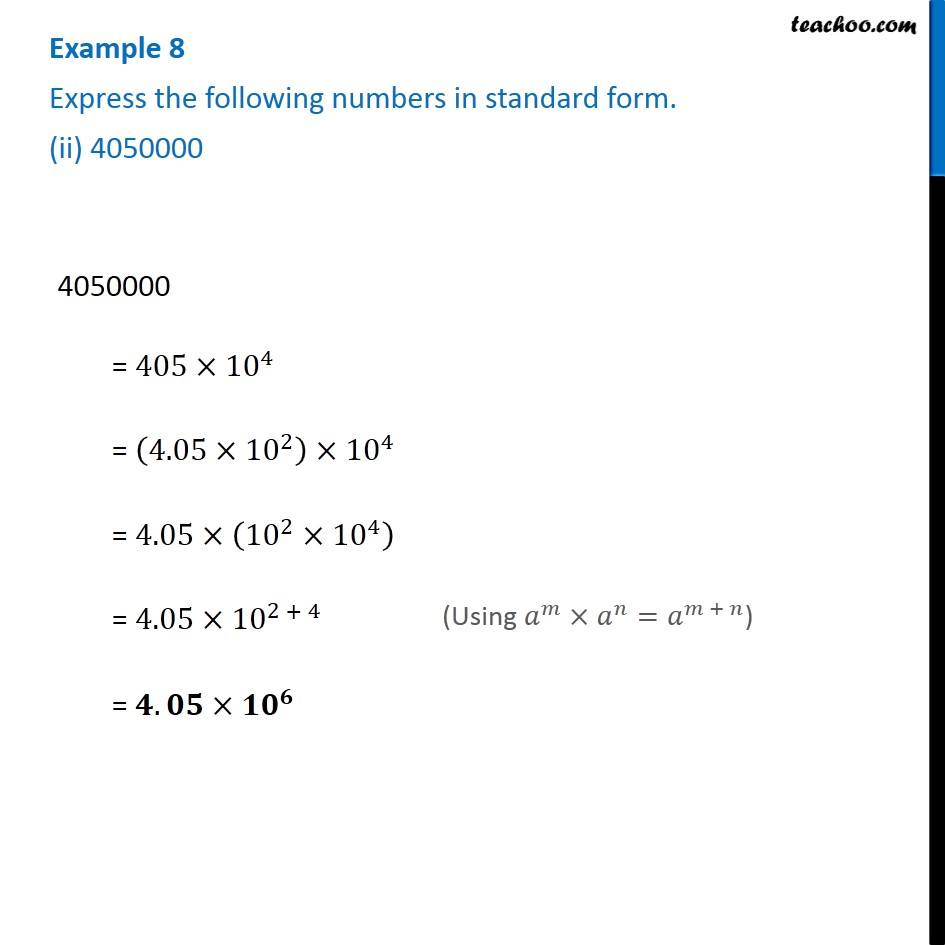1. Chapter 12 Class 8 Exponents and Powers
2. Serial order wise
3. Examples

Transcript

Example 8 Express the following numbers in standard form. (i) 0.000035 0.000035 = 35/1000000 = 35/〖10〗^6 = 35×〖10〗^(−6) = (3.5×10)×〖10〗^(−6) = 3.5×(10×〖10〗^(−6) ) = 3.5×〖10〗^(1 + (−6)) = 3.5×〖10〗^(1 − 6) = 𝟑.𝟓×〖𝟏𝟎〗^(−𝟓) (Using 𝑎^𝑚×𝑎^𝑛=𝑎^(𝑚 + 𝑛)) Example 8 Express the following numbers in standard form. (ii) 4050000 4050000 = 405×〖10〗^4 = (4.05×〖10〗^2 )×〖10〗^4 = 4.05×(〖10〗^2×〖10〗^4 ) = 4.05×〖10〗^(2 + 4) = 𝟒.𝟎𝟓×〖𝟏𝟎〗^𝟔 (Using 𝑎^𝑚×𝑎^𝑛=𝑎^(𝑚 + 𝑛))

Examples